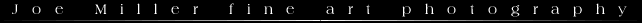NOTE:  In the near future I may discoutinue some of the javascript calculators on this site.  If you are interested in downloading a free border caculator go to http://www.fineart-photography.com/BorderCalc.html

Placing an Image Window at Absolute Center

To calculate the borders needed to center a window on a mat enter the measurements in the table below.  Those using inches will need to convert any fractions to decimals by using the "Fractions to Decimals" table.  The results can be converted back into fractions by using the javascript converter.  It will round to the nearest sixteenth of an inch which should be close enough for most purposes.

 1/16 = .0625 5/16 = .3125 9/16 = .5625 13/16 = .8125 2/16 or 1/8 = .1250 6/16 or 3/8 = .3750 10/16 or 5/8 = .6250 14/16 or 7/8 = .8750 3/16 = .1875 7/16 = .4375 11/16 = .6875 15/16 = .9375 4/16 or 1/4 = .2500 8/16 or 1/2 = .5000 12/16 or 3/4 = .7500

 Length of Mat Width of Mat Length of Window Width of Window Top & Bottom Border Side Borders Messages
Convert Decimal Fraction to Nearest Sixteenth of an Inch
Decimal Fraction: would be: sixteenths

Matting Prints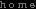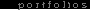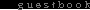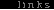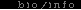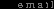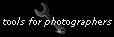All Text and Images © Joe Miller, 2004